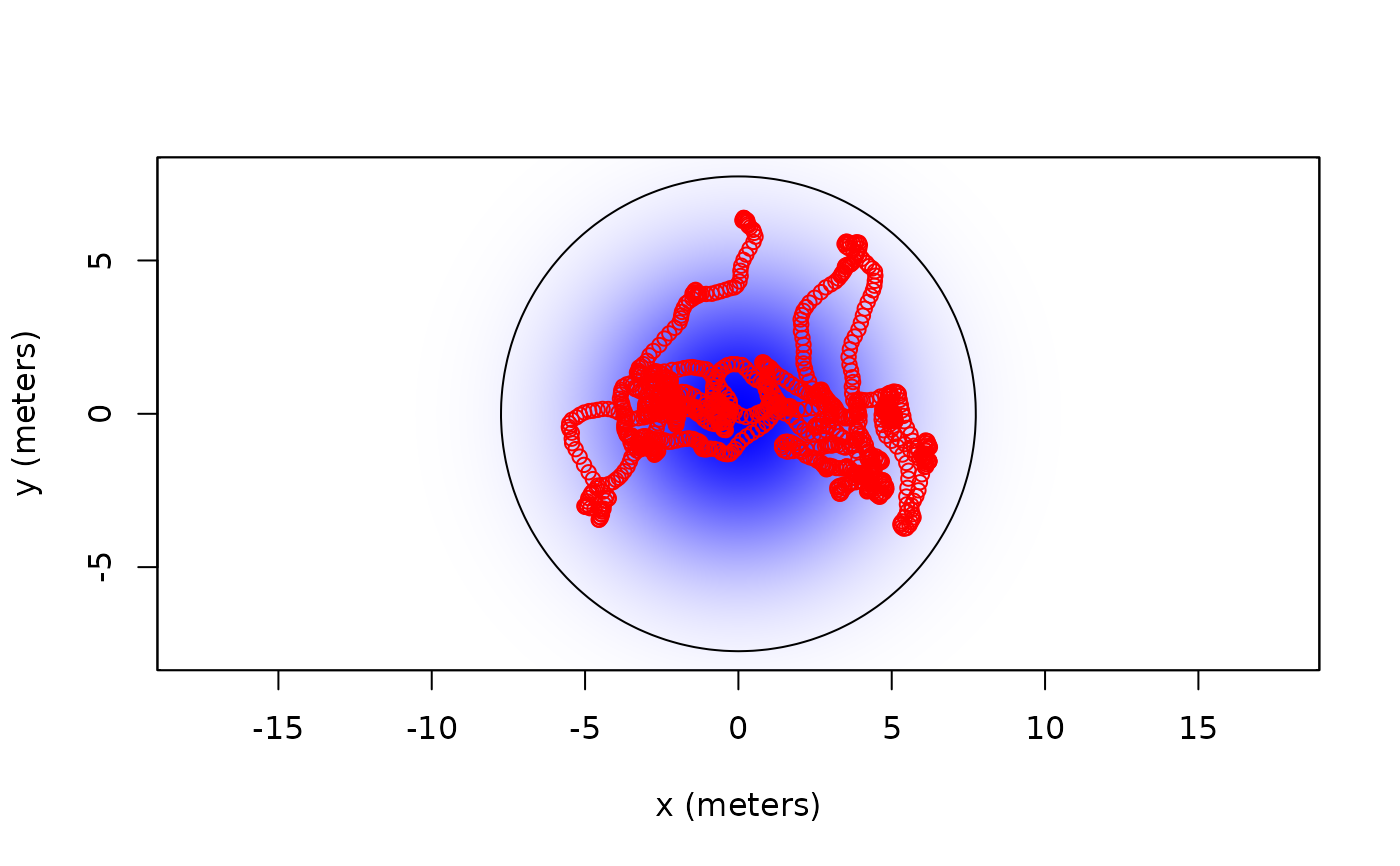Given a ctmm movement model (and optional telemetry data to condition upon) these functions predict or simulate animal locations over a prescribed set of times.

predict(object,...)

# S3 method for ctmm
predict(object,data=NULL,VMM=NULL,t=NULL,dt=NULL,res=1,complete=FALSE,...)

# S3 method for telemetry
predict(object,CTMM=NULL,VMM=NULL,t=NULL,dt=NULL,res=1,complete=FALSE,...)

simulate(object,nsim=1,seed=NULL,...)

# S3 method for ctmm
simulate(object,nsim=1,seed=NULL,data=NULL,VMM=NULL,t=NULL,dt=NULL,res=1,complete=FALSE,
precompute=FALSE,...)

# S3 method for telemetry
simulate(object,nsim=1,seed=NULL,CTMM=NULL,VMM=NULL,t=NULL,dt=NULL,res=1,complete=FALSE,
precompute=FALSE,...)

## Arguments

object A ctmm movement-model or telemetry object, which requires an additional CTMM argument. Optional telemetry object on which the prediction or simulation will be conditioned. A ctmm movement model in the same format as the output of ctmm.fit or variogram.fit. An optional vertical ctmm movement model for 3D predictions and simulations. Optional array of numeric time values over which the process will be predicted or simulated. Timestep to space the prediction or simulation over if data is specified. Average number of locations to predict or simulate per data time. Additionally calculate timestamps and geographic coordinates. Not yet supported. Optional random seed to fix. Precalculate matrices of the Kalman filter (see details). Unused options.

## Details

The prediction or simulation necessarily requires a ctmm model object. If a telemetry data object is supplied, the output will be conditional on the data (i.e., simulations that run through the data). If no data is provided then the output will be purely Gaussian, and times t must be provided. Details of the movement model parameters can be found in ctmm.fit.

The t argument fixes the output times to a specific array of times. The dt and res arguments are relative to the sampling schedule present in the optional telemetry object. The same span of time will be used, while dt will fix the sampling rate absolutely and res will fix the sampling rate relative to that of the data.

The precompute option can speed up calculations of multiple simulations of the same model, data, and irregular sampling schedule. First run simulate with precompute=TRUE to calculate and store all of the necessary matrices of the Kalman filter. A simulated telemetry object will be produced, as usual, and the precomputed objects are stored in the environment. Subsequent simulations with precompute=-1 will then apply these precomputed matrices for a computational cost savings. If the sampling schedule is irregular, then this can result in faster simulations.

## Value

A simulated animal-tracking telemetry object with components t, x, and y, or a predicted telemetry object that also includes x-y covariances for the location point estimates x and y.

C. H. Fleming, J. M. Calabrese, T. Mueller, K.A. Olson, P. Leimgruber, W. F. Fagan, From fine-scale foraging to home ranges: A semi-variance approach to identifying movement modes across spatiotemporal scales'', The American Naturalist, 183:5, E154-E167 (2014) doi: 10.1086/675504 .

C. H. Fleming, D. Sheldon, E. Gurarie, W. F. Fagan, S. LaPoint, J. M. Calabrese, Kálmán filters for continuous-time movement models'', Ecological Informatics, 40, 8-21 (2017) doi: 10.1016/j.ecoinf.2017.04.008 .

C. H. Fleming.

## Note

Predictions are autocorrelated and should not be treated as data.

ctmm.fit

## Examples

#Load package
library(ctmm)

#prepare simulation parameters
t <- 1:1000
MODEL <- ctmm(tau=c(100,10),sigma=10,mu=c(0,0))

#simulate data
SIM <- simulate(MODEL,t=t)

#plot data with Gaussian model
plot(SIM,CTMM=MODEL)